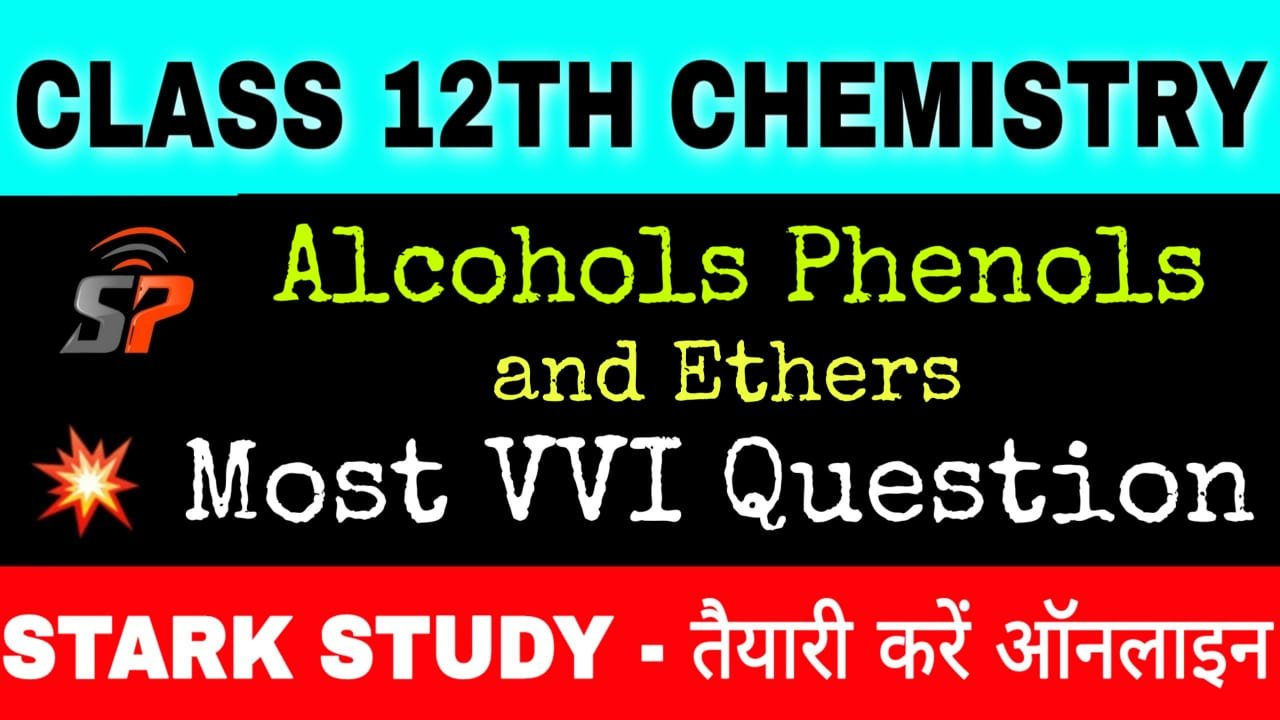BSEB 12th Exam Chemistry VVI Important Objective Question Chapter Wise

11. ALCOHOLS, PHENOLS AND ETHERS

1. Which of the following alcohols gives 2-butene on dehydration by conc. H2SO4

(A) 2-Methyl propene-2-ol

(B) 2-Methyl 1-propanol

(C) Butane-2-ol

(D) Butane 1-ol

2. The functional group of alcohol is :

(A) -COOH

(B) -CHO

(C) -C= O

(D) -OH

3. Alcohol is formed when one of the hydrogen of Alkane is replaced by :

(A) Hydroxyl group

(B) Aldehyde group

(C) Carboxylic group

(D) Chloro group

4. Which of the following is most acidic ?

(A) Benzyl alcohol

(B) Cyclohexanol

(C) Phenol

(D) m-Chlorophenol

5. The formula of Phenol is :

(A) C6H5OH

(B) C2H5OH

(C) C3H4OH

(D) C6H6

6. The I.U.P.A.C. name of CH3OC2H5 is

(A) Methoxy methane

(B) Ethoxy ethane

(C) Ethoxy methane

(D) None

7. The formula of methanol is :

(A) CH3OH

(B) CH3ONa

(C) HCHO

(D) CH4

8. The product obtained when silica reacts with hydrogen fluoride is :

(A) SiF4

(B) H2SiF6

(C) H2SIF4

(D) HASIF

9. Which of the following is a formula of methoxy methane ?

(A) CH3OCH3

(B) CH3COCH3

(C) C2H5OCH3

(D) C2H5COCH3

10. Formula of oleum is :

(A) H2S2O7

(B) H2SO4

(C) H2SO3

(D) H2SO5

11. Ethanol when heated with excess of conc. H2SO4 at 170° C gives :

(A) Ethane

(B) Ethene

(C) Dimethyl ether

(D) Ethyl hydrogen Sulphate

12. Ethanol is soluble in water because it forms :

(A) Ionic bond with water

(B) Covalent bond with water

(C) Hydrogen bond with water

(D) All of these